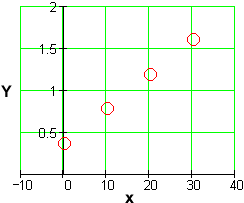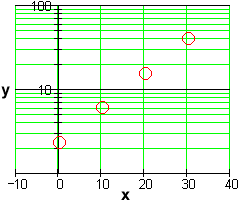Semi-Log Transformations of Data

Suppose a data set is actually following the trend of some hidden exponential function

y = a b x

If we take the logarithm of both sides of this equation (any logarithm will do) and use the laws of logarithms (see the section on algebraic representations of logarithms), we get

log(y) = log(a) + x log(b)

Now consider a new data set showing  Y = log(y)  vs.  x . If we let  A = log(a)  and  B = log(b) , then

Y = A + B x

This is linear. Linear data sets are easy to recognize.

The transformation of the data set from  y  vs.  x  to  Y = log(y)  vs.  x  is called a semi-log transformation. We take the logarithm of the data values in the output column of the data set (but not the input column – thus "semi") to discover the exponential trend. (Compare this with the log-log data transformations discussed in the section on numeric representations of power functions.)

For example, if we look again at the data set for  g , together with its semi-log transformation (we use  log = log 10), we have:

 x y 0 2.30 10 5.97 20 15.47 30 40.13

 x Y 0 0.36 10 0.78 20 1.19 30 1.60

Notice that we could not have made this transformation in the data set for  h , since the output values there are negative, and we cannot take logarithms of negative numbers.

It isn't difficult to recognize the linear trend in the transformed data set, with a slope of approximately  0.04  and a y-intercept of  0.36 . (See the section on numerical representations of linear functions.) We have  A = log(a) = 0.36  and  B = log(b) = 0.41 . Exponentiating gives  a = 10 0.36 = 2.3  and  b = 10 0.04 = 1.1 . Thus we may represent the original data set with the equation

g(x) = (2.3) (1.1) x

This is exactly the model we found using our previous methods.

There are also graphical versions of the semi-log data transformation.

The simplest is to plot  Y = log(y)  vs.  x  (rather than  y  vs.  x) and look for a straight line:The straight line tells us that the original data set has an exponential trend.

Alernatively, we can produce a semi-log plot of the original, untransformed data set  y  vs.  x  and look for a straight line:In this plot, the distances of the markings on the vertical scale above the x-axis are the logarithms of the distances used on a standard plot. The effect of the plot is to show the trends in the transformed data set without actually having to compute all of the logarithms.